• 毕业多年，曾经有同事问我该如何理解特征值的意义？当时，实在羞愧，我一学数学的，真不知该如何回答。极力回想，也只能以“特征值的求法、步骤...bla...bla...”应付了事，答非所问，简直了得！这样的答案教科书里...毕业多年，曾经有同事问我该如何理解特征值的意义？
当时，实在羞愧，我一学数学的，真不知该如何回答。
极力回想，也只能以“特征值的求法、步骤...bla...bla...”应付了事，
答非所问，简直了得！
人家问的是意义，如何理解其意义？
直扣灵魂，
我真的曾经理解过它的意义吗？？？
招了吧，真没有！
原在数学系时，教室里，对着黑板一堆密密麻麻的公式，我也是时常神游天外的主......
考试前，为了避免挂科才熬夜突击，对着书本一一比划，至少要演算两到三张稿纸，才能勉强记住方法、步骤，哪还管得着它的意义？
这种突击式的训练记忆，忘得也快，就像写代码一样，过一阵就忘了！
课堂上，老师大多是照本宣科。
当年，
也许是自己知识阅历不够，很难理解其意义，
也许是努力不够，被足球耽误了。
也许是天赋所致，不能顿悟！
...
总之，确定那时我肯定是没有理解它的意义的。
不知道现在有多少学生还是一样？
在学习一些抽象的数学工具时，代换三、四步之后就不知所云了，往往只能靠记忆强撑，而这种记忆最多维持一周，年轻时可能长点，后来，说忘就忘了......。
有极少数天才，能在抽象世界里面一直转，抽啊抽，一直抽......并最终以此为业。
而大多数人(99+%)，一到毕业，就尴尬，因为真的不理解其意义，
看似学了一些高深的数学知识，只会做题，不会运用，根本不理解公式指代符号的现实映射！进而职场上，其它方面训练缺失的短板逐渐显现后，囧是必然！
我想，这不单是数学教育的问题，也是其它各方面可能会尴尬的本源：
不理解意义！
好，扯远了，回到正题，来看灵魂之问：
如何理解特征值的意义？
最近才有些感悟，和大家分享一下。
说到特征值，数学上，基本上是指矩阵的特征值。
说到矩阵，高等代数几乎一整本书都在讲它，最著名的数学软件叫Matlab，直译为矩阵实验室，足见其高深、复杂！
而这么复杂混乱的东西确有一个特征值， 难道不奇怪？
再说，矩阵到底有多复杂混乱？看数学公式体会一下吧：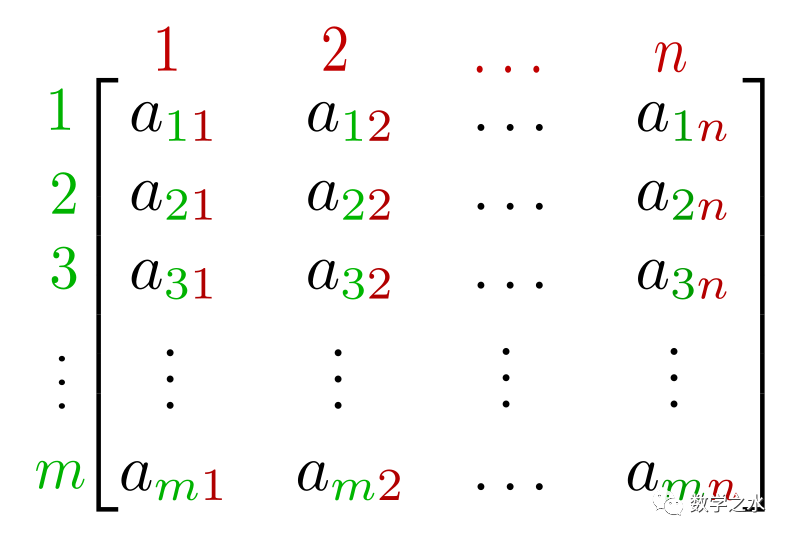这是一堆数，每一个数字都可以在实数域内取值(正、负、零)，或可以无限的延伸，联想到现在的大数据，还有什么东西不能由它表示呢？如果您相信万物皆数，这儿都可以说万物皆矩阵了，万物，能不复杂？
另外，这一堆数既可以表示数据记录，还可以表示某种不知名的抽象运算(物理上叫算子)，这样的数学运算，对某些对象集，确仅仅以固有的方式伸缩，且不管它是数据记录还是抽象运算，全都一样！
如此混乱复杂！ 确有本征!
这不神奇吗？
数学就是这样，抽象、高级、有理！
如果这样说感觉虚玄，那么先来看一下它精确(枯燥)的数学定义：

特征值
设是一矩阵，是一维非零列向量，若存在一数，使得

则称为的一个特征值，为的属于特征值的一个特征向量。
展开， 即

若把矩阵的每一行理解为一个基向量，则是表示基向量与该向量的内积() 等于。

感觉公式真的很枯燥的话，就先跳过上面吧。
下面我将从三个方面来试图阐释其意义，以便大家更好的理解。
几何上医学上物理上
(一)几何上
如果把矩阵理解为一个坐标系(不一定直角的，也可能是严重变形的“尺子”)，有一类向量叫特征向量，其中的任一个向量，在该矩阵上的投影，都只是自身固定的伸缩！
如何理解投影呢？且拿三维来理解吧，一根射线在另外一个坐标系(矩阵)下的影子，其每一轴都会有投影分量，把所有分量组合还原成影子，跟其自身共线，影子和射线的长度比值永远固定，这个比值就是特征值，简如下图。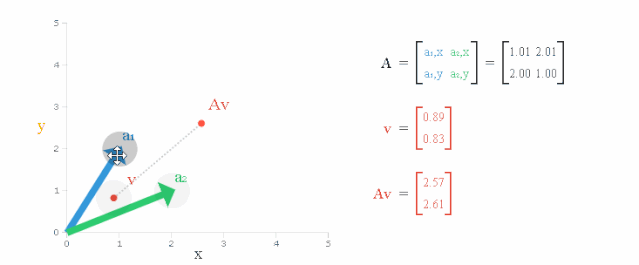而该比值对这条直线上的所有向量都适应，即无论射线长短。那么有多少条这样的直线呢？维矩阵最多有条，每一条的比值(特征值)可能都不一样，有大有小，都代表这一维度的自身特征，故这里大、小意义就明显了。
大可以理解为该维度上尺子的单位刻度大，比如表示一个单位刻度小可以理解为该维度上尺子的单位刻度小，比如表示一个单位刻度
(二) 医学上
如果把矩阵理解为中医祖传秘籍(乱不外传的)，特征向量理解为秘方子(枸杞、百合、红花、童子尿...)，特征值就是对该方子的用药量，温、热、寒不同方子特征值不一样， 这样也说得通，如下图！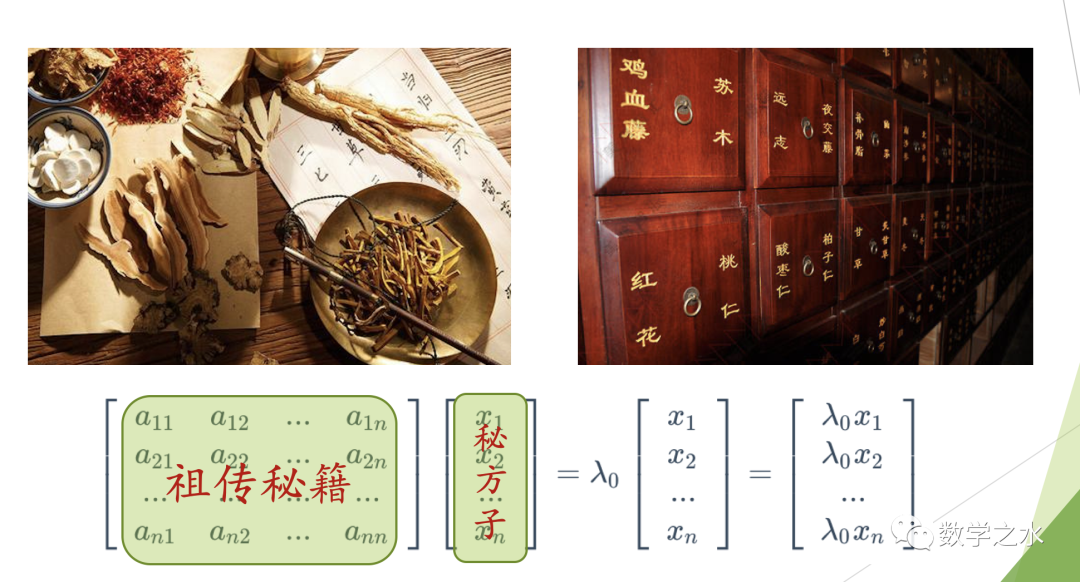进一步，把西药制成品也类比为特征向量。比如新冠治疗中的瑞得西韦， 特征值就是该神药该服用多少？还有其它药方子，如莲花清瘟等，假设都能治疗新冠肺炎，但用量肯定是不一样的，即不同特征向量对应的特征值不一。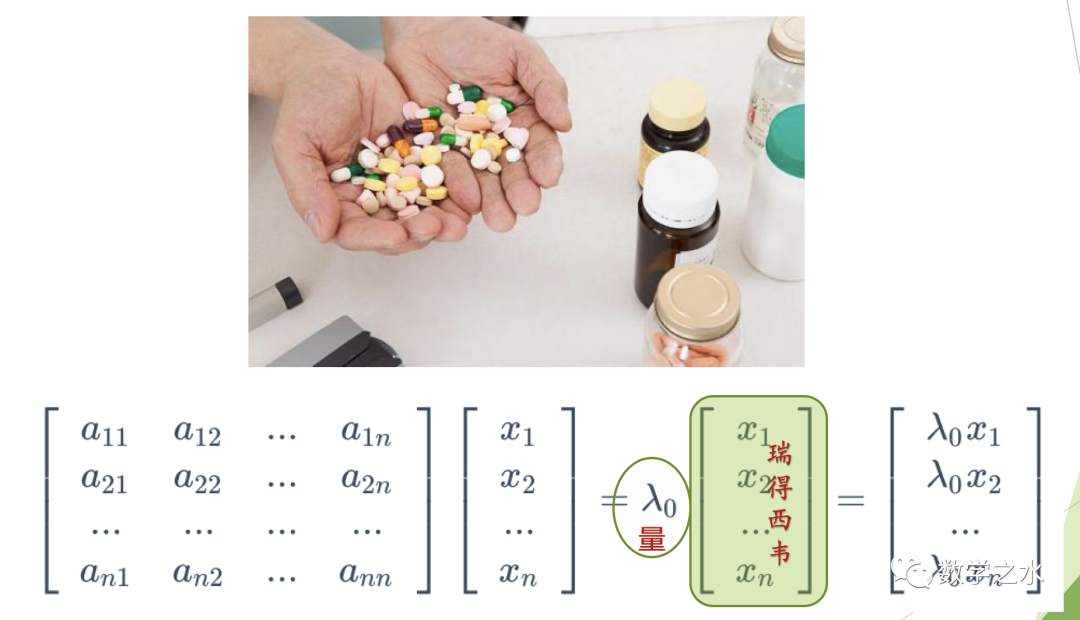如此看来，特征值可理解为医学上药物用量的一个刻度，也是中西医互相密而不宣的沟通桥梁，正如下图的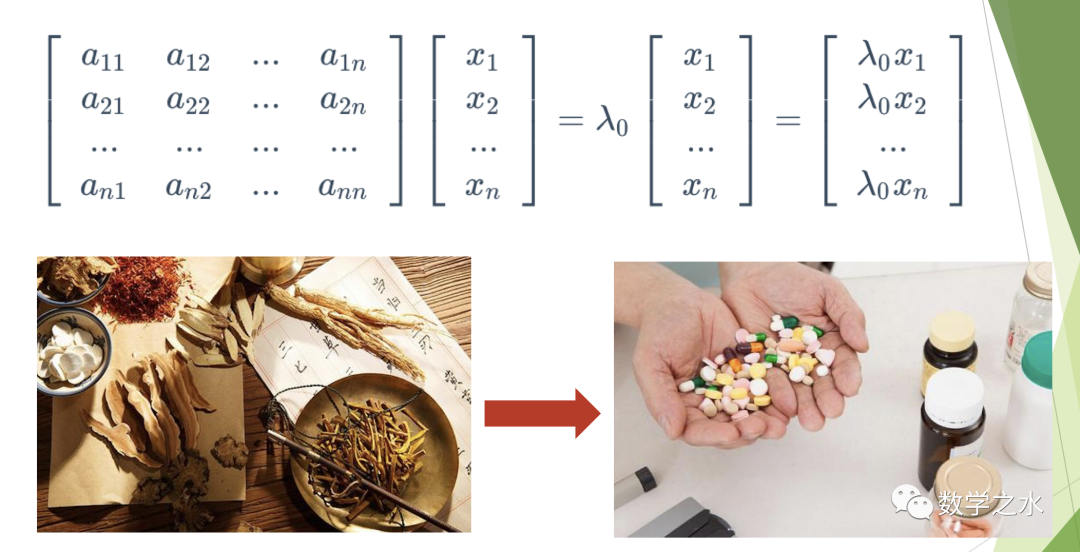(三) 物理上
“遇事不决，量子力学” 戏谑的表明了量子力学的高深、难懂！
且看薛定谔方程的前半部分，就复杂得都让人头晕眼花.....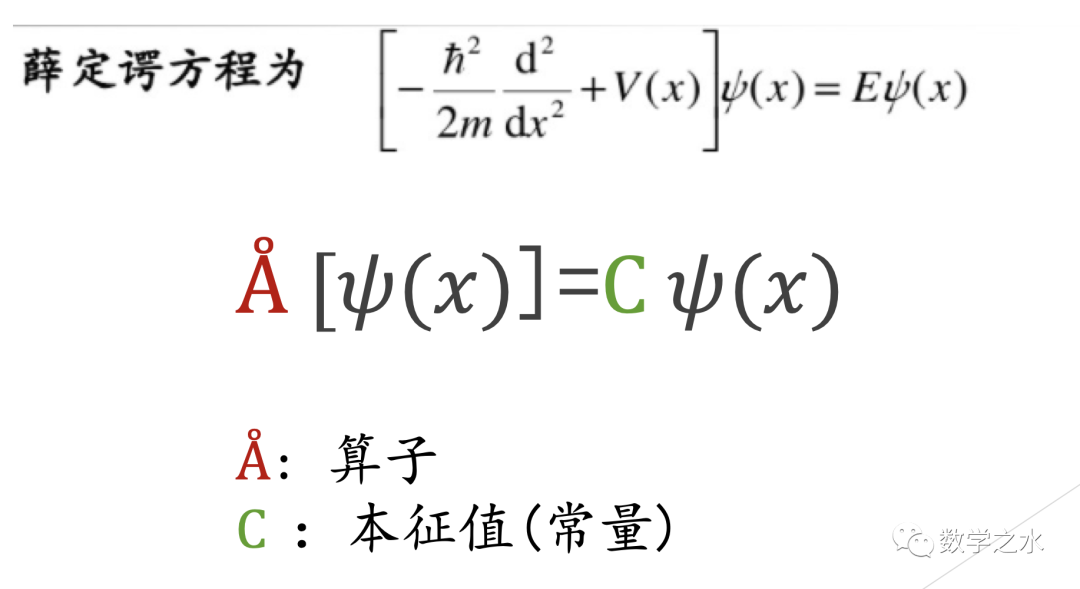物理学家把这种神操作统称为算子(因为给您解释不清楚~)，是不是有点巫师作法、道士占卜的感觉？
不同的是那帮巫师(物理学家)，在圈内对不同公式符号都给出了互相认可的解释！
例如：量子力学把世界看成是波动的，如果一个波函数经过一个量子变换后，它仍是一同一个波函数乘一个常量(如上图C)。
......
再看矩阵，它不也就是一个算子吗？而且还是线性的，如此简单，so easy!
大巫师(物理学家)牛！
这样，特征值的意义又从矩阵的线性上升到非线性统一了。
还是大巫师(物理学家)牛~
总之，就是一段复杂的操作，统称为算子，特征值也叫算子的本征值，台湾人习惯这样称呼，同一个意思，英文词源其实来自德语(自身的)。本来很好理解的概念，几经"转手"之后就晦涩难懂了....
遥想当年，若彼时能有这样的理解，就完美了！
想记这点感悟也很久了，
若有缘遇上，能给您带来一点点共鸣，便是满足。
最后附上特征值的求法，以便大家回忆。
附：特征值求法

特征多项式

它是数域上的一个次多项式，若是复数域，必有个根。每一个根都是矩阵的一个特征值
求特征值与特征向量方法步骤
求出特征多项式的全部根把所求根代入方程组中求出一组基础解系，就得到属于相应特征值的线性无关的特征向量

数学之水，更多免费干货、敬请关注详查~~展开全文• 特征值和特征向量（Eigenvalues and eigenvectors） 在线性代数中，一个线性变换的特征向量（eigenvector 或者 characteristic vector）是一个非零向量。将线性变换应用在它上面，它最多以一个标量因子进行伸缩变换...
特征值和特征向量（Eigenvalues and eigenvectors）
在线性代数中，一个线性变换的特征向量（eigenvector 或者 characteristic vector）是一个非零向量。将线性变换应用在它上面，它最多以一个标量因子进行伸缩变换。特征向量缩放的因子叫做特征值，记为

λ

\lambda

。
几何上，一个特征向量，对应于一个实非零特征值，指向它被变换拉伸的方向，特征值是它被拉伸的因子。如果特征值是负的，则方向相反，如果特征值为0，则缩回原点。
不严谨地说，在多维向量空间中，特征向量是不会旋转的。
正式定义
如果

T

T

一个线性变换，是一个从在 数域

F

F

上的向量空间

V

V

到它自身的映射。

v

\bold{v}

是一个在

V

V

内的非零向量。则

v

\bold{v}

是一个

T

T

的特征向量如果

T

(

v

)

T(\bold{v})

是

v

\bold{v}

的数乘（scalar multiple）。形式化地，

T

(

v

)

=

λ

v

,

T(\bold{v})=\lambda\bold{v},

其中，

λ

\lambda

是一个

F

F

内的标量，即特征值（eigenvalue或者characteristic value）。
由于存在

n

×

n

n\times n

的方阵 和 从

n

n

维向量空间到自身的线性变换之间直接的一一对应关系，而且是给定向量空间中的任意基的情况下。因此，在一个有限维的向量空间内，用矩阵的语言或者是线性变换的语言来定义特征值和特征向量是等价的。
如果

V

V

是有限维的，上述等式与

A

u

=

λ

u

A \bold{u} = \lambda \bold{u}

等价。其中

A

A

是

T

T

的矩阵表示，

u

\bold{u}

是

v

\bold{v}

的坐标向量（用坐标来表示的向量）。
概述（Overview）
特征值和特征向量在线性变换分析中占有突出地位。前缀 eigen- 取自德语单词 eigen（与英语单词 own 同源），表示“正确”、“特征”、“拥有”。特征值和特征向量最初用于研究刚体旋转运动的主轴，具有广泛的应用，例如稳定性分析、振动分析、原子轨道、面部识别和矩阵对角化。
此处的蒙娜丽莎示例提供了一个简单的说明。图像上的每个点都可以表示为从图像中心指向该点的向量。本例中的线性变换称为 shear 映射。上半部分的点向右移动，下半部分的点向左移动。指向原始图像中每个点的向量因此向右或向左倾斜，并通过变换变长或变短。应用此变换时，沿水平轴的点根本不会移动。因此，任何直接指向右侧或左侧且没有垂直分量的向量都是此变换的特征向量，因为映射不会改变其方向。此外，这些特征向量都有一个等于 1 的特征值，因为映射也不会改变它们的长度。在这个 shear 映射中，红色箭头改变方向，但蓝色箭头没有。蓝色箭头是此 shear 映射的特征向量，因为它不改变方向，并且由于其长度不变，因此其特征值为 1。

线性变换可以采用多种不同的形式，将向量映射到多种向量空间中，因此特征向量也可以采用多种形式。例如，线性变换可以是一个微分算子（differential operator），如

d

d

x

\frac{\mathrm{d} }{\mathrm{d} x}

。在这种情况下，特征向量是的函数，被称为特征函数（本征函数，eigenfunctions），特征向量是被微分算子scaled的，比如

d

d

x

e

λ

x

=

λ

e

λ

x

\frac{\mathrm{d} }{\mathrm{d} x}e^{\lambda x}=\lambda e^{\lambda x}

或者，线性变换可以采用

n

×

n

n × n

矩阵的形式，在这种情况下，特征向量是

n

×

1

n × 1

矩阵。如果线性变换以

n

×

n

n × n

矩阵

A

A

的形式表示，则上述线性变换的特征值方程可以重写为矩阵乘法

A

v

=

λ

v

A\bold{v}=\lambda \bold{v}

其中特征向量

v

\bold{v}

是一个

n

×

1

n × 1

矩阵。对于矩阵，特征值和特征向量可用于分解矩阵——例如通过对其进行对角化。
特征值和特征向量产生了许多密切相关的数学概念，并且在命名它们时可以随意使用前缀 eigen-：
线性变换的所有特征向量的集合，每个特征向量都与其对应的特征值配对，称为该变换的特征系统（eigensystem）。对应于相同特征值的

T

T

的所有特征向量的集合，加上零向量，称为特征空间（eigenspace），或被称为与该特征值相关的

T

T

的特征空间。如果

T

T

的一组特征向量形成

T

T

的domain基，则该基称为特征基（eigenbasis）。
历史
18 世纪，Leonhard Euler 研究了刚体的旋转运动，发现了主轴的重要性。Joseph-Louis Lagrange 意识到主轴是惯性矩阵的特征向量。（惯性张量的特征值和特征向量分别是主惯性矩和主惯性轴的方向）
在 19 世纪初，Augustin-Louis Cauchy 看到了他们的工作如何用于对二次曲面（quadric surfaces）进行分类，并将其推广到任意维度。柯西还创造了术语 racine caractéristique（特征根），即现在所谓的特征值；他的术语存在于特征方程中。
后来，约瑟夫·傅立叶在他 1822 年的著名著作《Théorie analytique de la chaleur》中，利用拉格朗日和皮埃尔-西蒙·拉普拉斯的工作，通过变量分离来求解热方程。Charles-François Sturm 进一步发展了傅立叶的思想，并引起了柯西的注意，柯西将它们与自己的思想结合起来，得出了实对称矩阵（real symmetric matrices）具有实特征值的事实。 1855 年，Charles Hermite 将其扩展为现在称为 Hermitian 矩阵。
大约在同一时间，Francesco Brioschi 证明了正交矩阵的特征值位于单位圆（unit circle）上，和 Alfred Clebsch 发现了反对称矩阵（斜对称矩阵，skew-symmetric matrices）的相应结果。最后，Karl Weierstrass 通过意识到亏损矩阵会导致不稳定，阐明了拉普拉斯提出的稳定性理论中的一个重要方面。
与此同时，Joseph Liouville 研究了类似于 Sturm 的特征值问题；从他们的工作中产生的学科现在被称为 Sturm-Liouville 理论。施瓦茨在 19 世纪末研究了拉普拉斯方程在一般域上的第一个特征值，而庞加莱在几年后研究了泊松方程。
20 世纪初，大卫希尔伯特通过将算子视为无限矩阵来研究积分算子的特征值。他是第一个使用德语单词 eigen 的人，意思是“拥有”，表示 特征值和特征向量，在1904年。尽管他可能一直在遵循赫尔曼·冯·亥姆霍兹的相关用法。一段时间以来，英语中的标准术语是“proper value”，但更独特的术语“eigenvalue”是今天的标准。
第一个计算特征值和特征向量的数值算法出现在 1929 年，当时 Richard von Mises 发表了power method。当今最流行的方法之一，QR 算法，由 John G. F. Francis 和 Vera Kublanovskaya 于 1961 年独立提出。
矩阵特征值和特征向量
特征值和特征向量通常在以矩阵为重点的线性代数课程中介绍给我们。此外，有限维向量空间上的线性变换可以使用矩阵来表示，这在数值和计算应用中尤其常见。矩阵 A 通过拉伸向量 x 而不改变其方向来起作用，因此 x 是 A 的特征向量

考虑形成为 n 个标量列表的 n 维向量，例如三维向量

x

=

[

1

−

3

4

]

y

=

[

−

20

60

−

80

]

\bold{x}=\begin{bmatrix} 1\\-3\\4 \end{bmatrix} \qquad \bold{y}=\begin{bmatrix} -20\\60\\-80 \end{bmatrix}

x

y

是相互的 标量倍数（scalar multiples），或平行或共线（parallel or collinear），如果有一个标量

λ

\lambda

使得

x

=

λ

y

\bold{x} = \lambda \bold{y}

在上面的例子中，

λ

=

−

1

20

\lambda = -\frac{1}{20}

.
现在考虑由

n

×

n

n × n

矩阵

A

A

定义的

n

n

维向量的线性变换，

A

v

=

w

A\bold{v}=\bold{w}

或者

[

A

11

A

12

⋯

A

1

n

A

21

A

22

⋯

A

2

n

⋮

⋮

⋮

A

n

1

A

n

2

⋯

A

n

n

]

[

v

1

v

2

⋮

v

n

]

=

[

w

1

w

2

⋮

w

n

]

\begin{bmatrix} A_{11}&A_{12}&\cdots A_{1n}\\ A_{21}&A_{22}&\cdots A_{2n}\\ \vdots & \vdots&\vdots\\ A_{n1}&A_{n2}&\cdots A_{nn} \end{bmatrix} \begin{bmatrix} v_{1}\\ v_{2}\\ \vdots \\ v_{n} \end{bmatrix}= \begin{bmatrix} w_{1}\\ w_{2}\\ \vdots \\ w_{n} \end{bmatrix}

对于每一行

w

i

=

A

i

1

v

1

+

A

i

2

v

2

+

⋯

+

A

i

n

v

n

=

∑

j

=

1

n

A

i

j

v

j

w_{i}=A_{i1}v_{1}+A_{i2}v_{2}+\dots+A_{in}v_{n}=\sum _{j=1}^{n}A_{ij}v_{j}

如果 存在

v

\bold{v}

和

w

\bold{w}

是标量倍数关系，即如果

A

v

=

w

=

λ

v

(1)

A\bold{v}=\bold {w}=\lambda \bold{v} \tag{1}

则

v

\bold{v}

是线性变换

A

A

的一个特征向量，标量因子

λ

\lambda

是特征值，对应于那个特征向量。方程（

1

\bold{1}

） 是矩阵

A

A

特征值方程。
方程（

1

\bold{1}

）可以等价地写成

(

A

−

λ

I

)

v

=

0

⃗

(2)

(A-\lambda I)\bold{v} = \vec{0} \tag{2}

其中

I

I

是

n

n

阶单位矩阵。
特征值和特征多项式（characteristic polynomial）
方程（

2

\bold{2}

）有一个非零解

v

v

当且仅当 矩阵

A

−

λ

I

A-\lambda I

的行列式是

0

0

。所以

A

A

的特征值是满足下面方程的

λ

\lambda

∣

A

−

λ

I

∣

=

0

(3)

|A-\lambda I|=0 \tag{3}

根据 行列式的定义（Leibniz’s rule），方程(

3

\bold{3}

) 是一个关于变量

λ

\lambda

的多项式函数，多项式的阶数是

n

n

（矩阵

A

A

）的阶数。多项式的系数取决于

A

A

，除了

n

n

阶的项总是

(

−

1

)

n

λ

n

(-1)^{n}\lambda ^{n}

。这个多项式被称作

A

A

的特征多项式。 方程(

3

\bold{3}

) 被称作

A

A

的特征方程（the characteristic equation or the secular equation）。
代数学基本定理（The fundamental theorem of algebra）指出

n

×

n

n\times n

矩阵的特征多项式，作为一个

n

n

阶多项式，可以被分解为

n

n

个线性项的乘积：

∣

A

−

λ

I

∣

=

(

λ

1

−

λ

)

(

λ

2

−

λ

)

…

(

λ

n

−

λ

)

,

(4)

|A-\lambda I|= (\lambda_{1}-\lambda)(\lambda_{2}-\lambda)\dots(\lambda_{n}-\lambda),\tag{4}

其中，

λ

i

\lambda_{i}

是复数域上的数。

λ

1

,

λ

2

,

…

λ

n

\lambda_{1},\lambda_{2},\dots\lambda_{n}

是多项式的根和矩阵

A

A

的特征值，不一定拥有不同的值。
作为一个简短的例子，在后面的例子部分更详细地描述，考虑矩阵

A

=

[

2

1

1

2

]

A= \begin{bmatrix} 2&1\\1&2 \end{bmatrix}

取

(

A

−

λ

I

)

(A − λI)

的行列式，

A

A

的特征多项式为

∣

A

−

λ

I

∣

=

[

2

−

λ

1

1

2

−

λ

]

=

3

−

4

λ

+

λ

2

|A-\lambda I|=\begin{bmatrix} 2-\lambda &1\\ 1&2-\lambda \end{bmatrix} =3-4\lambda+\lambda^{2}

令特征多项式为零，它的根为

λ

=

1

λ=1

和

λ

=

3

λ=3

，这是

A

A

的两个特征值。每个特征值对应的特征向量可以通过求解方程

(

A

−

λ

I

)

v

=

0

{\displaystyle \left(A-\lambda I\right)\mathbf {v} =\mathbf {0} }

得到。在这个例子中，特征向量是

v

λ

=

1

=

[

1

−

1

]

,

v

λ

=

3

=

[

1

1

]

的任何数乘向量。
如果矩阵 A 的元素都是实数，那么特征多项式的系数也将是实数，但特征值可能仍然有非零虚部（nonzero imaginary parts）。因此，相应特征向量的元素也可能具有非零虚部。类似地，即使

A

A

的所有元素都是有理数，或者即使它们都是整数，特征值也可能是无理数。
具有实系数的实多项式的非实根可以分组为复共轭对，即每对复共轭的两个成员具有仅符号不同的虚部和相同的实部。如果阶数是奇数，则根据intermediate value 定理，根中至少有一个是实数。因此，任何奇数阶实矩阵至少有一个实特征值，而偶数阶实矩阵可能没有任何实特征值。与复数特征值相关联的特征向量也是复数并且也出现在复共轭对中。
代数重数（Algebraic multiplicity）
令

λ

i

\lambda_{i}

为

n

×

n

n\times n

矩阵

A

A

的一个特征值。特征值的代数重数

μ

A

(

λ

i

)

\mu_{A}(\lambda_{i})

是其作为特征多项式根的多重性，即最大整数

k

k

使得

(

λ

−

λ

i

)

k

(λ − λi)^k

整除该多项式。
假设矩阵

A

A

具有维度

n

n

，且

d

≤

n

d ≤ n

个不同的特征值。而等式

(

4

)

(4)

将

A

A

的特征多项式分解为

n

n

个线性项的乘积，其中一些项可能重复，而特征多项式可以写为 d 项的乘积，每个项对应于一个不同的特征值，每个项的指数是代数重数，

∣

A

−

λ

I

∣

=

(

λ

1

−

λ

)

u

A

(

λ

1

)

(

λ

1

−

λ

)

u

A

(

λ

2

)

…

(

λ

1

−

λ

)

u

A

(

λ

d

)

|A-\lambda I| = (\lambda_{1}-\lambda)^{u_{A}(\lambda_{1})}(\lambda_{1}-\lambda)^{u_{A}(\lambda_{2})}\dots(\lambda_{1}-\lambda)^{u_{A}(\lambda_{d})}

如果

d

=

n

d=n

那么 右式是

n

n

个线性项，上式就和等式（

4

\bold4

）一样了。每个特征值的代数重数大小和 维度

n

n

有下列关系

1

≤

u

A

(

λ

i

)

≤

n

u

A

=

∑

i

=

1

d

u

A

(

λ

i

)

=

n

1 \le u_{A}(\lambda_{i}) \le n \\ u_{A} = \sum_{i=1}^{d}u_A(\lambda_{i})=n

如果

u

A

(

λ

i

)

=

1

u_A(\lambda_{i})=1

，那么

λ

i

\lambda_{i}

被称作一个simple特征值。 如果

u

A

(

λ

i

)

u_A(\lambda_{i})

和

λ

i

\lambda_{i}

的几何重数（在下一section介绍）相等，那么

λ

i

\lambda_{i}

被称作 semisimple 特征值。
特征空间，几何重数，和矩阵的特征基（Eigenspaces，geometric multiplicity，and the eigenbasis for matrices）
给定

n

×

n

n × n

矩阵

A

A

的特定特征值

λ

λ

，将集合

E

E

定义为满足方程 (

2

\bold2

) 的所有向量

v

\mathbf{v}

，

E

=

{

v

:

(

A

−

λ

I

)

v

=

0

⃗

}

E=\{\mathbf{v}:(A-\lambda I)\mathbf{v}=\vec{0}\}

一方面，这个集合是矩阵

(

A

−

λ

I

)

(A − λI)

的核或零空间。另一方面，根据定义，任何满足此条件的非零向量都是与

λ

λ

关联的

A

A

的特征向量。因此，集合

E

E

是零向量与与

λ

λ

关联的

A

A

的所有特征向量的集合的并集，并且 集合

E

E

等于

(

A

−

λ

I

)

(A − λI)

的零空间。

E

E

称为与

λ

λ

相关的

A

A

的特征空间（eigenspace ，characteristic space）。一般来说，

λ

λ

是一个复数，特征向量是复数

n

×

1

n × 1

矩阵。零空间的一个性质是它是一个线性子空间，所以

E

E

是

C

n

\mathbb{C}^{n}

的一个线性子空间。
因为特征空间

E

E

是一个线性子空间，所以它在加法下是封闭的。也就是说，如果两个向量

u

\mathbf{u}

和

v

\mathbf{v}

属于集合

E

E

，记作

u

,

v

∈

E

\mathbf{u}, \mathbf{v} ∈ E

，则

u

+

v

∈

E

\mathbf{u}+\mathbf{v} ∈ E

或等效地

A

(

u

+

v

)

=

λ

(

u

+

v

)

A(u + v) = λ(u + v)

。这可以使用矩阵乘法的分配特性(distributive property)来验证。类似地，因为

E

E

是一个线性子空间，它在标量乘法下是封闭的。也就是说，如果

v

∈

E

\mathbf{v} ∈ E

并且

α

\alpha

是一个复数，则

(

α

v

)

∈

E

(\alpha \mathbf{v}) ∈ E

或等效地

A

(

α

v

)

=

λ

(

α

v

)

A(\alpha \mathbf{v}) = λ(\alpha \mathbf{v})

。这可以通过注意到复数矩阵乘以复数是可交换的来检查。只要

u

+

v

\mathbf{u + v}

和

α

v

α\mathbf{v}

不为零，它们也是与

λ

λ

关联的

A

A

的特征向量。
与

λ

λ

相关联的特征空间

E

E

的维数，或等效地与

λ

λ

相关联的线性无关特征向量的最大数量，被称为特征值的几何重数

γ

A

(

λ

)

γA(λ)

。因为

E

E

也是

(

A

−

λ

I

)

(A − λI)

的零空间，

λ

λ

的几何重数是

(

A

−

λ

I

)

(A − λI)

的零空间的维度，也称为

(

A

−

λ

I

)

(A − λI)

的零空间

γ

A

(

λ

)

=

n

−

r

a

n

k

(

A

−

λ

I

)

γA(λ)=n-rank(A-\lambda I)

注：不同的特征向量可以对应同一个特征值，或者说同一个特征值可以对应多个特征向量。因为特征向量是和矩阵对应的，一个矩阵表示一个线性变换。特征向量就是在这次线性变换中只伸缩，不旋转的向量。特征值就是这次线性变换作用在这个特征向量上时，这个特征向量的伸缩倍数。所以多个不同的特征向量是可以伸缩同样的倍数的，即可以有相同的特征值。
特征值的其他性质

令

A

是

一

个

任

意

的

n

×

n

复

数

矩

阵

，

其

特

征

值

为

λ

1

,

…

,

λ

n

。

令 A 是一个任意的 n\times n 复数矩阵，其特征值为 \lambda _{1},\ldots,\lambda _{n}。

每个特征值在这个列表中出现

μ

A

(

λ

i

)

\mu _{A}(\lambda _{i})

次，其中

μ

A

(

λ

i

)

\mu _{A}(\lambda _{i})

是特征值的代数重数。以下是该矩阵及其特征值的属性：

A

A

的迹，定义为其对角线元素之和，也是所有特征值之和

t

r

(

A

)

=

∑

i

=

1

n

a

i

i

=

∑

i

=

1

n

λ

1

+

λ

2

+

⋯

+

λ

n

tr(A)=\sum_{i=1}^{n}a_{ii}=\sum_{i=1}^{n}\lambda_{1}+\lambda_{2}+\dots+\lambda_{n}

A 的行列式是其所有特征值的乘积，

∣

A

∣

=

∏

i

=

1

n

λ

i

|A|= \prod_{i=1}^{n}\lambda_i

矩阵

A

A

的

k

k

（k为正整数）次幂

A

k

A^{k}

的特征值为

λ

1

k

,

…

,

λ

n

k

\lambda_1^k,\dots,\lambda_n^k

。  矩阵

A

A

可逆当且仅当 它的每个特征值都非零。  如果

A

A

是可逆的，那么

A

−

1

A^{-1}

的特征值为

1

λ

1

,

…

,

1

λ

n

{\frac {1}{\lambda _{1}}},\ldots ,{\frac {1}{\lambda _{n}}}

， 并且每个特征值的几何重数对应相等。此外，由于逆的特征多项式是原多项式的reciprocal polynomial，特征值共享相同的代数重数。  如果

A

A

等于它的共轭转置

A

∗

A^{*}

，或者等价地，如果

A

A

是 Hermitian，那么每个特征值都是实数。这同样适用于任何对称实矩阵。  如果

A

A

不仅是 Hermitian，而且还是正定、正半定、负定或负半定，则每个矩阵的特征值分别为正、非负、负或非正。  如果

A

A

是幺正（unitary）的，每个特征值都有绝对值

∣

λ

i

∣

=

1

|\lambda _{i}|=1

。  如果

A

A

是一个

n

×

n

n\times n

矩阵和

{

λ

1

,

…

,

λ

k

}

\{\lambda _{1},\ldots ,\lambda _{k}\}

是它的特征值，那么矩阵

I

+

A

{\displaystyle I+A}

的特征值（其中

I

I

是单位矩阵）是

{

λ

1

+

1

,

…

,

λ

k

+

1

}

\{\lambda _{1}+1, \ldots ,\lambda _{k}+1\}

。此外，如果

α

∈

C

\alpha \in \mathbb {C}

，

α

I

+

A

\alpha I+A

的特征值是

{

λ

1

+

α

,

…

,

λ

k

+

α

}

\{\lambda _{1}+\alpha ,\ldots , \lambda _{k}+\alpha \}

。更一般地，对于多项式

P

P

，矩阵

P

(

A

)

P(A)

的特征值是

{

P

(

λ

1

)

,

…

,

P

(

λ

k

)

}

\{P(\lambda _{1}),\ldots ,P(\lambda _{k})\}

。
左右特征向量
许多学科传统上将向量表示为具有单列的矩阵，而不是具有单行的矩阵。因此，矩阵上下文中的“特征向量”一词几乎总是指右特征向量，即右乘

n

×

n

n\times n

矩阵

A

A

的列向量定义方程，方程（

1

\mathbf1

）

A

v

=

λ

v

A\mathbf{v}=\lambda \mathbf{v}

特征值和特征向量问题也可以定义为左乘矩阵$A$的行向量。在这个公式中，定义方程是:

u

A

=

κ

u

\mathbf{u}A = \kappa\mathbf{u}

其中

κ

\kappa

是一个标量，而且

u

\mathbf{u}

是一个

1

×

n

1\times n

的矩阵。任何满足上述方程的 行向量

u

\mathbf{u}

被称作 矩阵

A

A

的左特征向量，

κ

\kappa

被称作与左特征向量相关的特征值。对上述方程取转置，得：

A

T

u

T

=

κ

u

T

A^{T}\mathbf{u}^{T}=\kappa\mathbf{u}^{T}

将此等式与等式 (

1

\mathbf{1}

) 进行比较，可以立即得出

A

A

的左特征向量与

A

T

A^{\textsf {T}}

具有相同的特征值。此外，由于 $A^{\textsf {T}}$ 的特征多项式与

A

A

的特征多项式相同，所以

A

A

的左特征向量的特征值与

A

T

A^{\textsf {T}}

的右特征向量的特征值相同。
对角化和特征分解（Diagonalization and the eigendecomposition）
假设

A

A

的特征向量形成一个基，或者等价地，

A

A

有

n

n

个线性无关的特征向量

v

1

,

v

2

,

.

.

.

,

v

1

n

v_{1}, v_{2}, ..., v_{1n}

以及相关的特征值

λ

1

,

λ

2

,

.

.

.

,

λ

n

λ_{1}, λ_{2}, ..., λ_{n}

。特征值不需要是不同的。定义一个方阵

Q

Q

，其列是

A

A

的

n

n

个线性无关的特征向量，

Q

=

[

v

1

,

v

2

…

v

n

]

Q=[\mathbf{v}_{1},\mathbf{v}_{2}\dots\mathbf{v}_{n}]

由于

Q

Q

的每一列都是

A

A

的特征向量，因此将

A

A

乘以

Q

Q

将

Q

Q

的每一列按其关联的特征值缩放，

A

Q

=

[

λ

1

v

1

λ

2

v

2

…

λ

n

v

n

]

考虑到这一点，定义一个对角矩阵

Λ

\Lambda

，其中每个对角元素

Λ

i

j

\Lambda_{ij}

是与

Q

Q

的第

i

i

列相关联的特征值。然后

A

Q

=

Q

Λ

AQ=Q\Lambda

因为

Q

Q

的列是线性无关的，所以

Q

Q

是可逆的。等式两边右乘

Q

−

1

Q^{-1}

A

=

Q

Λ

Q

−

1

A=Q\Lambda Q^{-1}

或者等式两边左乘

Q

−

1

Q^{-1}

，得

Q

−

1

A

Q

=

Λ

Q^{-1}AQ=\Lambda

因此，

A

A

可以分解为由其特征向量组成的矩阵、特征值沿对角线的对角矩阵以及特征向量矩阵的逆矩阵。这称为特征分解(eigendecomposition)，它是一种相似变换。这样的矩阵

A

A

被称为相似于对角矩阵

Λ

Λ

或可对角化的。矩阵

Q

Q

是相似变换的基矩阵的变更（change of basis matrix of the similarity transformation）。本质上，矩阵

A

A

和

Λ

Λ

代表以两个不同基表示的相同线性变换。当将线性变换表示为

Λ

Λ

时，特征向量用作基。
相反，假设矩阵

A

A

是可对角化的。设

P

P

是一个非奇异方阵，使得

P

−

1

A

P

P^{-1}AP

是某个对角矩阵

D

D

。左乘

P

P

，

A

P

=

P

D

AP = PD

。因此，

P

P

的每一列必须是

A

A

的特征向量，其特征值是

D

D

的对应对角元素。由于

P

P

的列必须线性无关才能使

P

P

可逆，因此

A

A

存在

n

n

个线性无关的特征向量。进而，

A

A

的特征向量形成一个基当且仅当 A 是可对角化的。
不可对角化的矩阵称为亏损的(defective)。对于有亏损的矩阵，特征向量的概念推广到广义特征向量，特征值的对角矩阵推广到 Jordan 范式。在代数闭域上，任何矩阵 A 都具有 Jordan 范式，因此允许广义特征向量的基和分解为广义特征空间。
惯性张量
采用直角坐标系的三个坐标轴为参考轴，一个刚体的惯性张量

I

{\mathcal {I}}

，以矩阵形式表达为

[

I

x

x

I

x

y

I

x

z

I

y

x

I

y

y

I

y

z

I

z

x

I

z

y

I

z

z

]

\begin{bmatrix} I_{xx} & I_{xy} & I_{xz} \\ I_{yx} & I_{yy} & I_{yz} \\ I_{zx} & I_{zy} & I_{zz}\end{bmatrix}

其中，矩阵的元素以方程表达为

I

x

x

=

d

e

f

∫

y

2

+

z

2

d

m

I

x

y

=

I

y

x

=

d

e

f

−

∫

x

y

d

m

I

y

y

=

d

e

f

∫

x

2

+

z

2

d

m

I

x

z

=

I

z

x

=

d

e

f

−

∫

x

z

d

m

I

z

z

=

d

e

f

∫

x

2

+

y

2

d

m

I

y

z

=

I

z

y

=

d

e

f

−

∫

y

z

d

m

(

x

,

y

,

z

)

(x,\ y,\ z)

是刚体内部的微小体积元

d

m

dm

的位置。惯性张量

I

\mathcal{I}

是个实值三阶对称矩阵，对角元素

I

x

x

,

I

y

y

,

I

z

z

I_{xx},I_{yy},I_{zz}

分别为刚体对于 x-轴， y-轴，z-轴的转动惯量。非对角元素

I

α

β

，

α

≠

β

I_{\alpha \beta}， \alpha \ne \beta

是刚体对于

α

\alpha

-轴和

β

\beta

-轴的惯量积。根据谱定理，可以使惯性张量成为一个对角矩阵。所得到的三个特征值必定是正实值；三个特征向量必定正交。
换另外一种方法，我们需要求解特征方程

I

ω

=

λ

ω

\mathcal{I}\ \boldsymbol{\omega}=\lambda\;\boldsymbol{\omega}

也就是以下行列式等于零的三次方程：

∣

I

x

x

−

λ

I

x

y

I

x

z

I

y

x

I

y

y

−

λ

I

y

z

I

ω

=

λ

ω

z

x

I

z

y

I

z

z

−

λ

∣

=

0

\begin{vmatrix} I_{xx} - \lambda & I_{xy} & I_{xz} \\ I_{yx} & I_{yy} - \lambda & I_{yz} \\\mathcal{I}\ \boldsymbol{\omega}=\lambda\;\boldsymbol{\omega}_{zx} & I_{zy} & I_{zz} - \lambda \end{vmatrix}=0

这方程的三个根

λ

1

λ

2

λ

3

\lambda_1\,\lambda_2\,\lambda_3\,

都是正实的特征值。将特征值带入特征方程，再加上方向余弦（directional cosine）方程，

ω

x

2

+

ω

y

2

+

ω

z

2

=

1

\omega_x^2+\omega_y^2+\omega_z^2=1\,

。就可以求到特征向量

ω

^

1

ω

^

2

ω

^

3

\hat{\boldsymbol{\omega}}_1\,\hat{\boldsymbol{\omega}}_2\,\hat{\boldsymbol{\omega}}_3\,

。这些特征向量都是刚体的惯量主轴；而这些特征值则分别是刚体对于惯量主轴的主转动惯量。
其他
特征值和特征向量在PCA中的应用请移步PAC、SVD以及它们的联系之充分数学知识推导因子分析、图的特征值、特征脸、应力张量薛定谔方程、分子轨域、振动分析中特征值和特征向量的应用请移步参考文献1或者2。
参考文献
Eigenvalue and Eigenvector from wiki特征值和特征向量 来自维基百科两个特征值相等则特征向量是否相等？来自知乎
展开全文线性代数
• 6.1 矩阵的特征向量特征值 犹如世界上每个人都有自己的特点一样，每个矩 阵也有其内在的特性。可逆性、秩、初等变换的结果等属于矩阵的代数性质，而特征值、特征向量偏向于反映矩阵的几何特性。 A是n阶矩阵，x是n...
6.1 矩阵的特征向量与特征值
犹如世界上每个人都有自己的特点一样，每个矩 阵也有其内在的特性。可逆性、秩、初等变换的结果等属于矩阵的代数性质，而特征值、特征向量偏向于反映矩阵的几何特性。
A是n阶矩阵，x是n维列向量，则

A

x

Ax

也是n维列向量，当然它己经改变了原来的

x

x

的大小与方向。有没有一个特别的非零向量

x

x

，使得向量

A

x

Ax

仅仅使向量x伸长了若干倍而没有改变其方向呢？这个使

A

x

=

λ

x

Ax=\lambda x

成立的特别的向量因矩阵A而定，反映A的内在特性，故称之为特征向量，相应的数称为特征值。
特征值和特征向量的应用
特征向量不仅在数学上很重要，在物理，材料，力学等方面都能一展拳脚，s.J.Leon在一本线性代数书里这样说“特征值在我们生活中非常普遍，只要有振动就有特征值（振动的自然频率）。如果你曾经弹过吉他，你就求解了一个特征值问题"。
美国1940年建造了塔科马海峡桥，开始这座桥有小的振动，很多人好奇的在这座移动的桥上驾驶汽车，大约4个月后振动变大，最后这座桥坠落水中·这是由于风的频率接近这座桥的固有频率引起了共振，而这座桥的固有频率是桥的建模系统的绝对值最小的特征值。这就是特征值对于工程师分析建筑物的结构时非常重要的原因。一个工程师总是试图使他的桥梁或他的火箭的自然频率远离风的频率或液体燃料的频率；而在另一种极端情况，一个证券经纪人则努力去接近市场的自然频率线。
汽车的设计者研究特征值是为了抑制噪音从 而创造一个安静的乘车环境。石油公司借助特征值分析可以找到石油储藏 地点。特征值也可以用用于检查固体的裂缝，当一根量被撞击，它的固有频率（特征值）能够被听到，如果这根梁有回响表明他没有裂缝；如果声音迟钝，则这根梁有裂缝。用收音机收听广播时要改变谐振频率直到它与正在广播的频相匹配，因此设计收音机时要利用特征值。
定义：设A为n阶方阵，若存在数

λ

\lambda

及非零向量x使

A

x

=

λ

x

Ax=\lambda x

，则称数

λ

\lambda

为A的特征值，x为A的对应于

λ

\lambda

的特征向量。 例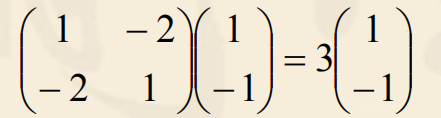注：
只有方阵才有特征向量；特征值使非零列向量；对应于同一特征值的特征向量有无穷多。
分析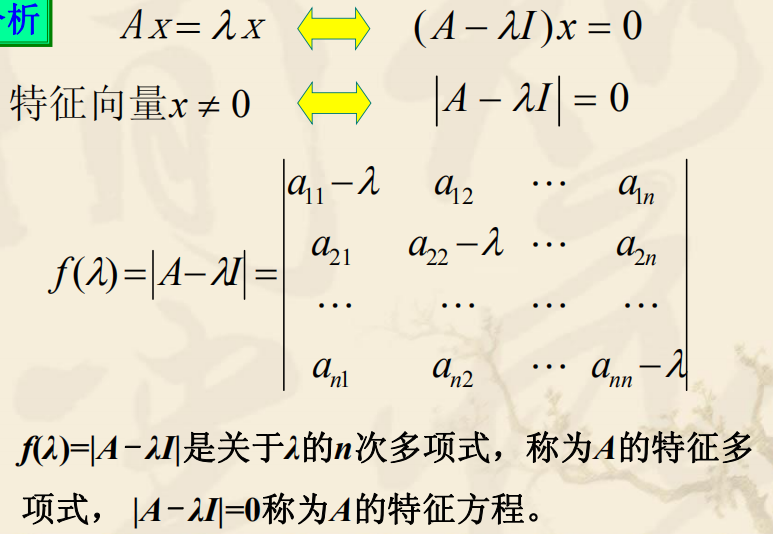代数学基本定理
一个n次复系数多项式方程在复数域内有且只有n 个根（重根按重数计算）。 关于代数学基本定理的证明，现有200多种证法。 第一个严格证明是高斯给出的。 例 求矩阵

A

=

(

1

0

0

0

2

0

0

0

3

)

A=\begin{pmatrix} 1 &0 &0 \\ 0 &2 &0 \\ 0 &0 &3 \end{pmatrix}

的特征值。

∣

A

−

λ

I

∣

=

∣

1

−

λ

0

0

0

2

−

λ

0

0

0

3

−

λ

∣

=

(

1

−

λ

)

(

2

−

λ

)

(

3

−

λ

)

\left | A-\lambda I \right |=\begin{vmatrix} 1-\lambda &0 &0 \\ 0 &2-\lambda &0 \\ 0 &0 &3-\lambda \end{vmatrix}=(1-\lambda)(2-\lambda)(3-\lambda)

λ

1

=

1

,

λ

2

=

2

,

λ

3

=

3

\lambda_{1}=1,\lambda_{2}=2,\lambda_{3}=3

对角矩阵

{

λ

1

λ

2

.

.

.

λ

n

}

\begin{Bmatrix} \lambda_{1} & & & \\ &\lambda_{2} & & \\ & &... & \\ & & &\lambda_{n} \end{Bmatrix}

的特征值就是主对角矩阵。 例 求矩阵

A

=

{

−

2

1

1

0

2

0

−

4

1

3

}

A=\begin{Bmatrix} -2 &1 &1 \\ 0 &2 &0 \\ -4 &1 &3 \end{Bmatrix}

的特征值和特征向量。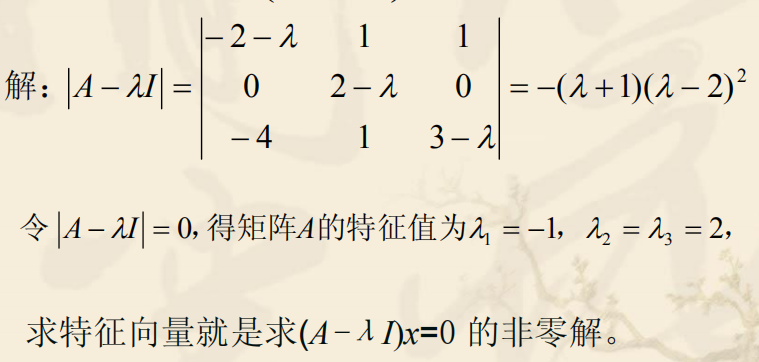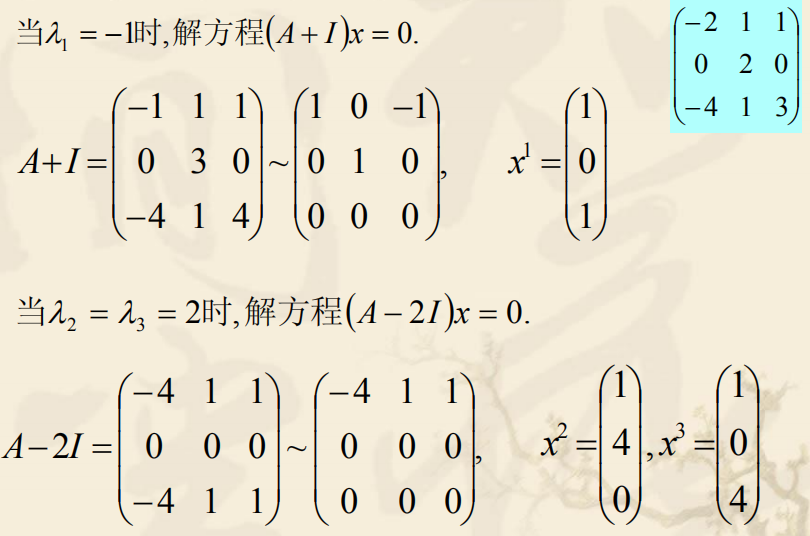例

A

=

{

1

2

2

2

1

−

2

−

2

−

2

1

}

A=\begin{Bmatrix} 1 & 2&2 \\ 2& 1 & -2\\ -2&-2 & 1 \end{Bmatrix}

解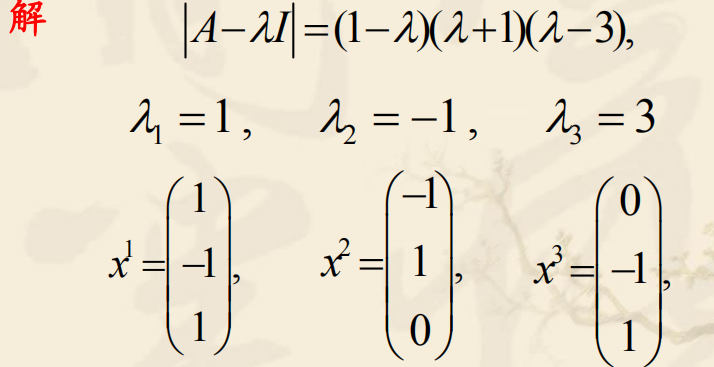练习 求下列矩阵的特征值和特征向量

A

=

(

0

0

1

0

1

0

1

0

0

)

A=\begin{pmatrix} 0 & 0&1 \\ 0& 1 & 0\\ 1&0 & 0 \end{pmatrix}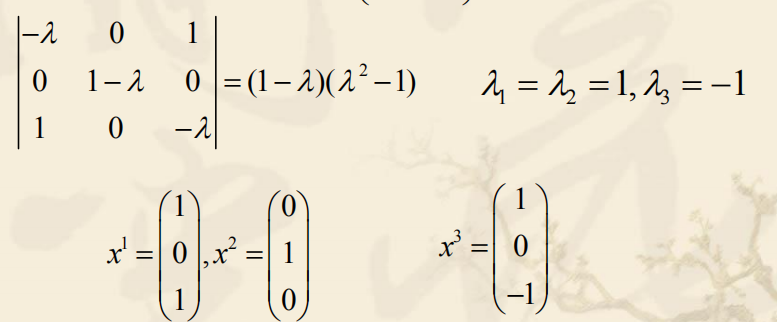特征值和特征向量的性质
定理1 若

λ

1

,

λ

2

,

.

.

.

,

λ

n

\lambda_{1},\lambda_{2},...,\lambda_{n}

为方阵A的n个特征值，则： (i)

λ

1

λ

2

.

.

.

λ

n

=

?

∣

A

∣

;

\lambda_{1}\lambda_{2}...\lambda_{n}=?|A|;

(ii)

λ

1

+

λ

2

+

.

.

+

λ

n

=

a

11

+

a

22

+

.

.

.

+

a

n

n

\lambda_{1}+ \lambda_{2}+..+\lambda_{n}=a_{11}+a_{22}+...+a_{nn}

定理2 方阵A的对应于不同特征值的特征向量线性无关。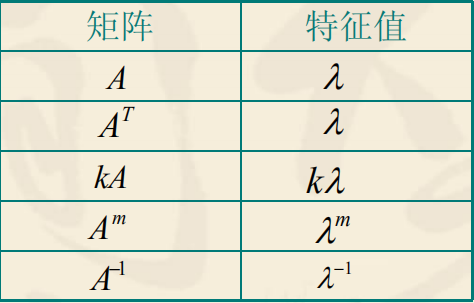若

λ

\lambda

是 A 的一个特征值，则

f

(

λ

)

=

a

0

+

a

1

+

λ

+

.

.

.

+

a

m

λ

m

f(\lambda)=a_{0}+a_{1}+\lambda+...+a_{m}\lambda_{m}

是矩阵多项式

f

(

A

)

=

a

0

I

+

a

1

A

+

.

.

.

+

a

m

A

m

f(A)=a_{0}I+a_{1}A+...+a_{m}A^{m}

的特征值。 例 设λ是方阵 A 的特征值，对应的特征向量为x。 证明： (1)

k

λ

k\lambda

是

k

A

kA

的特征值，对应的特征向量仍为x。 (2)

λ

2

\lambda^{2}

是

A

2

A^{2}

的特征值。 (3) 当 A 可逆时，

λ

−

1

\lambda^{-1}

是

A

−

1

A^{-1}

的特征值，对应的特征向量仍为x。 证 （1）

A

x

=

λ

x

;

(

k

A

)

x

=

(

k

λ

)

x

Ax=\lambda x;(kA)x=(k\lambda)x

（2）

A

2

=

A

(

A

x

)

=

A

(

λ

x

)

=

λ

(

A

x

)

=

λ

(

λ

x

)

=

λ

2

x

A^{2}=A(Ax)=A(\lambda x)=\lambda(Ax)=\lambda(\lambda x)=\lambda^{2}x

（3）

A

−

1

(

A

x

)

=

A

−

1

(

λ

x

)

=

λ

A

−

1

x

;

A

−

1

x

=

1

λ

x

A^{-1}(Ax)=A^{-1}(\lambda x)=\lambda A^{-1}x;A^{-1}x=\frac{1}{\lambda}x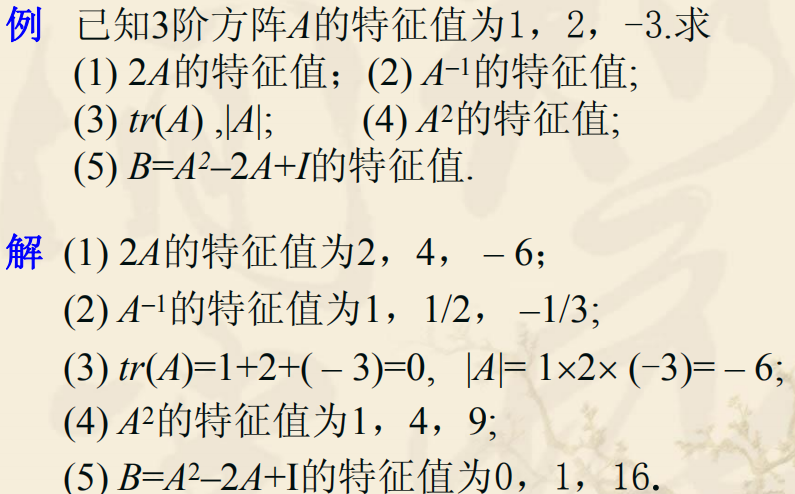练习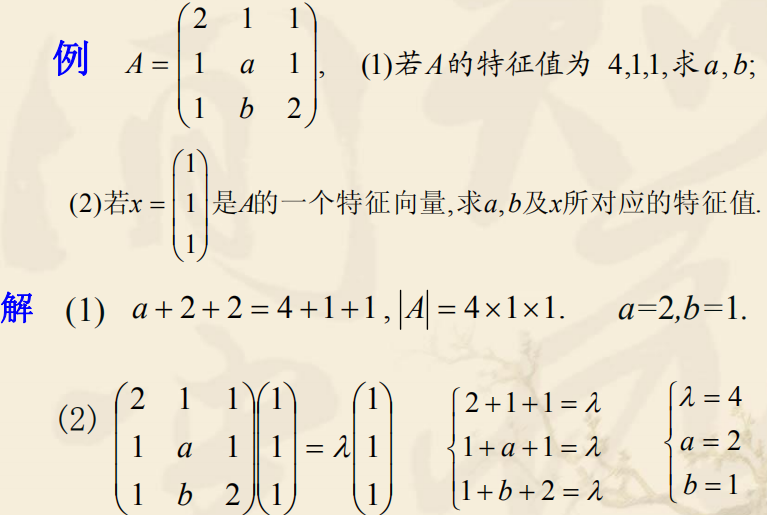6.2 相似矩阵与对角化
定义 设A、B为n阶方阵，若存在可逆矩阵P 使

P

−

1

A

P

=

B

P^{-1}AP=B

， 称矩阵A与B相似。特别地，如果A与对角矩阵相似, 称A可对角化。 相似矩阵的性质
相似的矩阵一定等价，反之不对。相似的矩阵有相同的特征值，从而有相同的行列式。若A与B相似，则

A

−

1

A^{-1}

与

B

−

1

B^{-1}

，

A

m

A^{m}

与

B

m

B^{m}

也相似。
下面讨论对角化的问题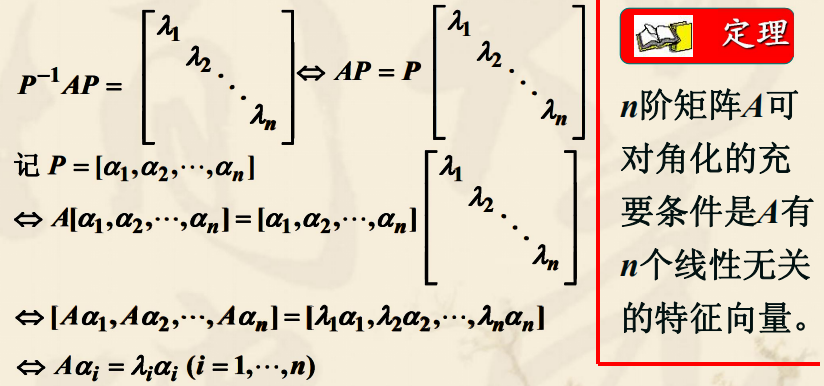这说明：如果A可对角化，它必有n个线性无关的特征向量， 就是P的n个列；反之，如果A有n个线性无关的特征向量，把它 拼成矩阵P(可逆)，把上面过程逆过来即知A可对角化。
推论 若n阶方阵A有n个不同的特征值, 则A可对角化。注意，并不是所有的方阵都能对角化，例如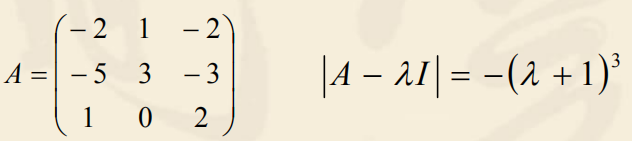所以A的特征值为

λ

1

=

λ

2

=

λ

3

=

−

1

\lambda_{1}=\lambda_{2}=\lambda_{3}=-1

。 把

λ

=

−

1

\lambda=-1

代入

(

A

−

λ

I

)

x

=

0

(A-\lambda I)x=0

, 解得基础解系

x

=

(

1

,

1

,

−

1

)

T

x=(1,1,-1)^{T}

, 只有一个线性无关的特征向量，故 A不能对角化。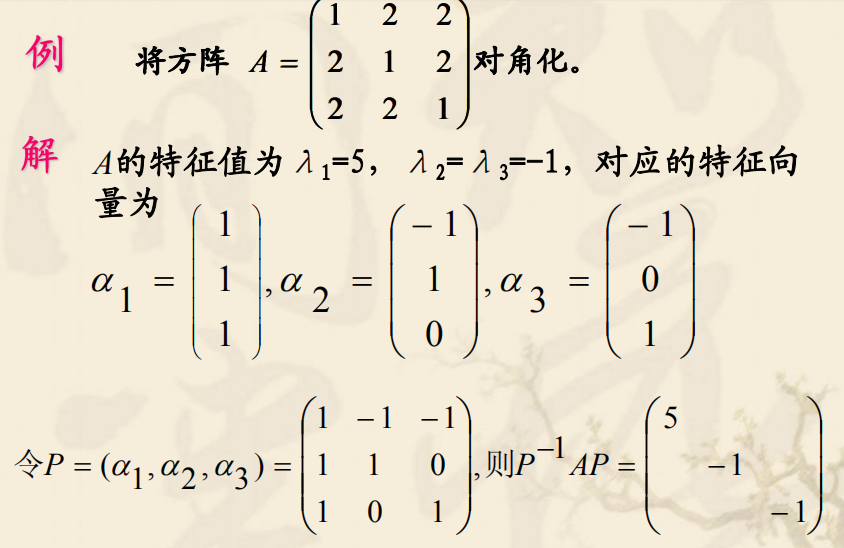6.3 实对称矩阵的对角化
对称矩阵：

A

=

A

T

A=A^{T}

正交矩阵：

A

T

=

A

−

1

等

价

于

A

A

T

=

I

A^{T}=A^{-1}等价于AA^{T}=I

性质
实对称矩阵的特征值是实数。实对称矩阵属于不同特征值的特征向量必正交。实对称矩阵一定可以对角化，且存在正交阵 Q， 使得Q −1AQ = QTAQ = D。 其中 D 是以 A 的 n 个特征值为对角元的对角阵。
用正交矩阵化A为对角阵的步骤： (1) 由

∣

A

−

λ

I

∣

=

0

|A-\lambda I|=0

求出A的全部特征值

λ

1

,

λ

2

,

.

.

.

,

λ

n

\lambda_{1},\lambda_{2},...,\lambda_{n}

; (2) 对每个λi ,求方程组

(

A

−

λ

i

I

)

x

=

0

(A-\lambda_{i}I)x=0

的基础解系, 即为A的属于特征值λi 的线性无关特征向量; (3) 将线性无关特征向量正交化、单位化，共可得到 n个两两正交的单位特征向量 q1, q2, … , qn; (4) 令 Q=(q1, q2, … , qn), 则Q为正交矩阵，且

Q

−

1

A

Q

=

Q

T

A

Q

=

D

Q^{-1}AQ=Q^{T}AQ=D

6.3 实对称矩阵的对角化
对称矩阵：

A

=

A

T

A=A^{T}

正交矩阵：

A

T

=

A

−

1

等

价

于

A

A

T

=

I

A^{T}=A^{-1}等价于AA^{T}=I

性质：
实对称矩阵的特征值是实数；实对称矩阵属于不同特征值的特征向量；实对称矩阵一定可以对角化，且存在正交阵 Q， 使得Q −1AQ = QTAQ = D. 其中 D 是以 A 的 n 个特征值为对角元的对角阵。
用正交矩阵化A为对角阵的步骤： (1) 由

∣

A

−

λ

I

∣

=

0

|A-\lambda I|=0

求出A的全部特征值 λ1, λ2,…, λn; (2) 对每个λi ,求方程组

∣

A

−

λ

I

∣

x

=

0

|A-\lambda I|x=0

的基础解系, 即为A的属于特征值λi 的线性无关特征向量; (3) 将线性无关特征向量正交化、单位化，共可得到 n个两两正交的单位特征向量 q1, q2, … , qn; (4) 令 Q=(q1, q2, … , qn), 则Q为正交矩阵，且

Q

−

1

A

Q

=

Q

T

A

Q

=

D

Q^{-1}AQ=Q^{T}AQ=D

。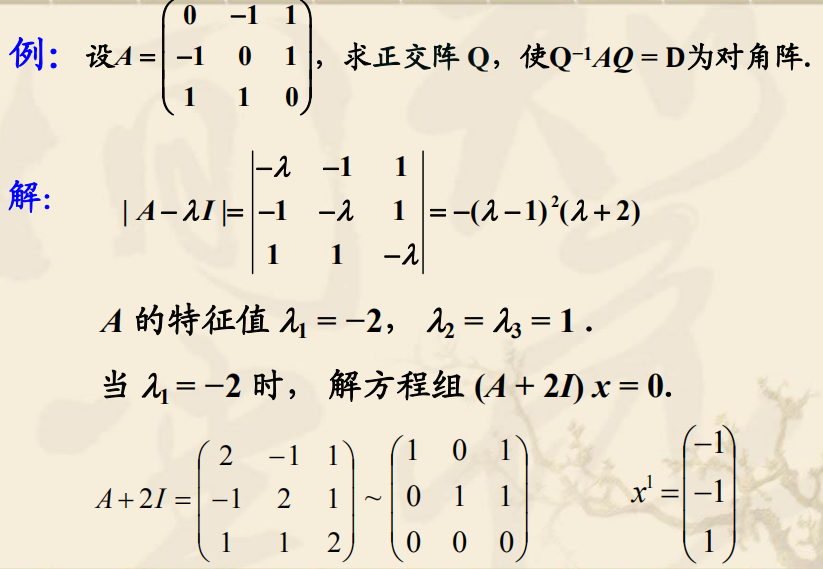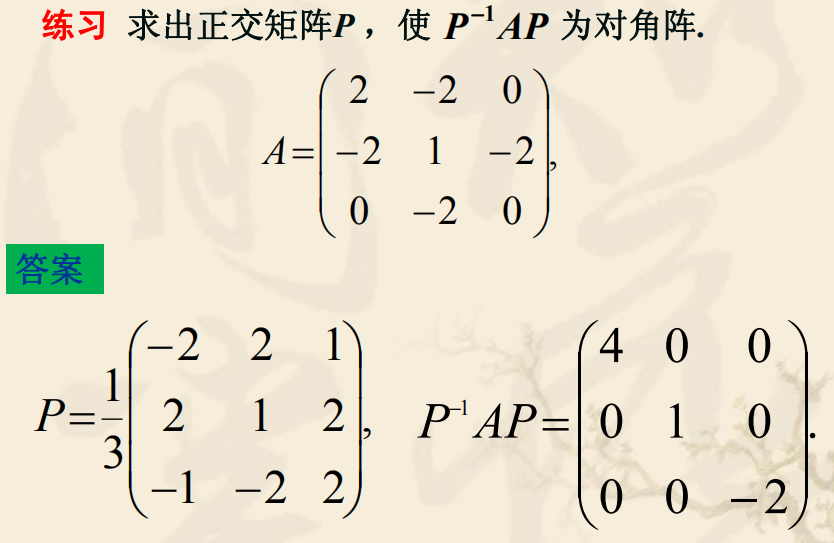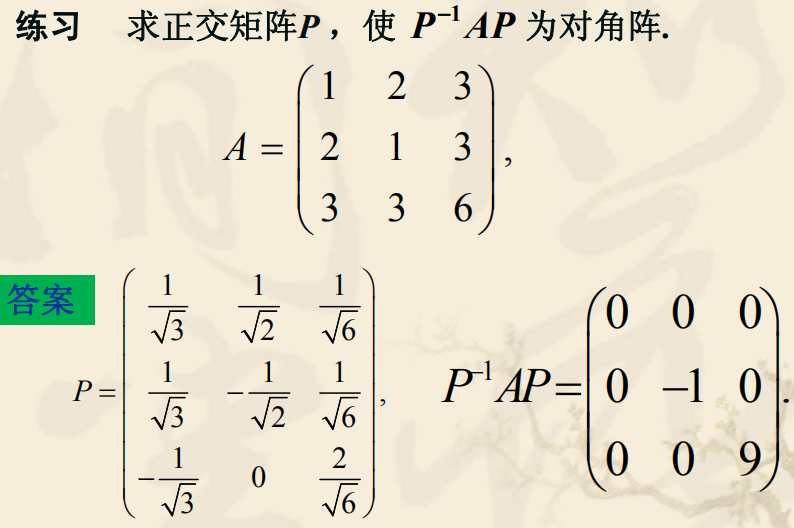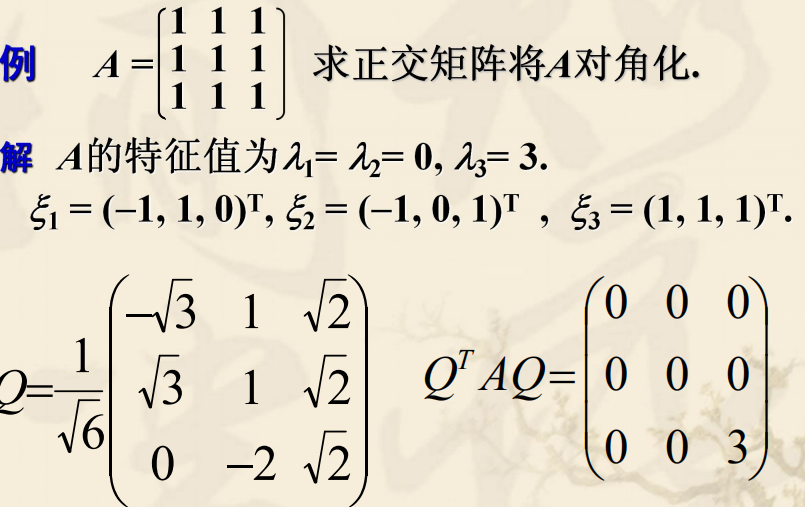展开全文• （个人观点，如有错漏请提出）抽象理解特征值（eigenvalue）和特征向量（eigenvector）具有共同前缀 eigen- ，其起源于德语，意为“特征”。首先我们应该充分理解“特征”的含义：对于线性代数而言，特征向量和特...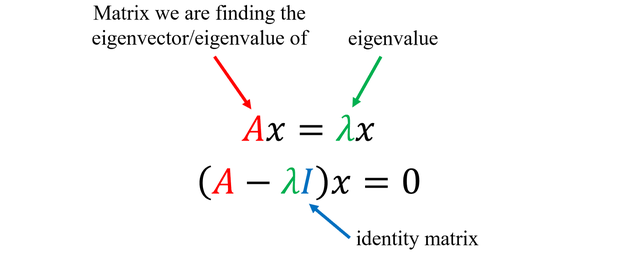Greeting！
特征值与特征向量是大学线性代数与统计学课程里的内容，当年强背了过去，并没有真正理解过这个问题。为了以后学习统计学习方法更方便，在此记录下学习文章以加深理解。（个人观点，如有错漏请提出）

抽象理解
特征值（eigenvalue）和特征向量（eigenvector）具有共同前缀 eigen- ，其起源于德语，意为“特征”。首先我们应该充分理解“特征”的含义：对于线性代数而言，特征向量和特征值体现了矩阵的本质，“特征”强调了单个矩阵的特点，相当于它的ID card。
从线性代数的角度出发，如果把矩阵看作n维空间下的一个线性变换，这个变换有很多的变换方向，我们通过特征值分解得到的前N个特征向量，那么就对应了这个矩阵最主要的N个变化方向。我们利用这前N个变化方向，就可以近似这个矩阵（变换）。其中的N个变化方向，就是这个矩阵最重要的“特征”。
有了特征的概念后，我们又如何理解特征值与特征向量呢？可以作这样比喻：
如果把矩阵看作是位移，那么特征值 = 位移的速度，特征向量 = 位移的方向。特征向量在一个矩阵的作用下作伸缩运动，伸缩的幅度由特征值确定（注意观察定义式）。特征值大于1，所有属于此特征值的特征向量变长；特征值属于(0, 1)，特征向量缩短；特征值小于0，特征向量则反向延长。
我们都知道线性代数中，左乘一个矩阵是对应着行变换，右乘一个矩阵对应列变换，其实际的作用也就是对常规坐标系进行了迁移。那么，【重点】对于在普通二维坐标系下的向量，它在矩阵描述空间中的表示与自己单纯的进行拉伸或者缩放的效果一致，满足这种特殊性的就是特征矩阵，对应的拉伸量就是特征值。

有了这个特殊的性质，特征向量与特征值出现在很多有矩阵运算的地方，如主成分分析（PCA）、奇异值分解（SVD）等机器学习方法中更是时常提到。至于PCA与SVD的基本思想，请看我接下来的科普文章。
关于定义
设A是n阶矩阵，如果存在常数和n维非零向量X，

使得则称为矩阵A的一个

特征值（标量），X为矩阵A对应于特征值的一个
特征向量（）

该式子可理解为向量x在几何空间中经过矩阵A的变换后得到向量。由此可知，向量经过矩阵A变换后，只是大小伸缩了倍。总而言之：

特征向量提供了复杂的矩阵乘法到简单的数乘之间的转换！
并且，我们有以下推论：其中第三个是特征值分解公式，为的

特征向量矩阵（n个大小为的特征向量组成）。是包含对应特征值的

对角矩阵。根据不同的特征值的大小，可以知道每个特征向量对应权重，即其重要性。
从解题的角度，我们再来谈谈如何求特征值和特征向量：
设向量a为矩阵A对应于特征值 λ 的特征向量，
即则有：所以求解a就是求解中的非零解。

其中I是单位矩阵，因此称为A的

特征多项式。

前置知识
（1）方差、协方差、相关系数、协方差矩阵
方差
方差用来度量随机变量 X 与其数学期望 E(X) 的偏离程度，公式为：方差总是一个非负数，当随机变量的可能值集中在数学期望的附近时，方差较小；反之方差大。由方差的大小可以推断随机变量分布的分散程度。
协方差
协方差用来刻画两个随机变量 X , Y 的相关性，公式为：如果协方差为正，说明X，Y同向变化，协方差越大说明同向程度越高；如果协方差为负，说明X，Y反向运动，协方差越小说明反向程度越高。 对上述“同向”和“反向”的理解： 1）你变大，同时我也变大，说明两个变量是同向变化的，这时协方差就是正的。 2）你变大，同时我变小，说明两个变量是反向变化的，这时协方差就是负的。 3）从数值来看，协方差的数值越大，两个变量同向程度也就越大。反之亦然。
相关系数
用随机变量X，Y的协方差除以X的标准差和Y的标准差，公式为：相关系数也可以看成是协方差：一种剔除了两个变量量纲，标准化后的协方差。
相关系数是一种标准化后的协方差，有以下特点： 1）也可以反映两个变量变化时是同向还是反向，如果同向变化就为正，反向变化就为负。 2）它消除了两个变量变化幅度的影响，而只是单纯反应两个变量每单位变化时的相似程度。
对于两个随机变量： 1）当他们的相关系数为1时，说明两个变量变化时的正向相似度最大，即，你变大一倍，我也变大一倍；你变小一倍，我也变小一倍。也即是完全正相关（以X、Y为横纵坐标轴，可以画出一条斜率为正数的直线，所以X、Y是线性关系的）。 2）随着他们相关系数减小，两个变量变化时的相似度也变小，当相关系数为0时，两个变量的变化过程没有任何相似度，也即两个变量无关。 3）当相关系数继续变小，小于0时，两个变量开始出现反向的相似度，随着相关系数继续变小，反向相似度会逐渐变大。 4）当相关系数为－1时，说明两个变量变化的反向相似度最大，即，你变大一倍，我变小一倍；你变小一倍，我变大一倍。也即是完全负相关（以X、Y为横纵坐标轴，可以画出一条斜率为负数的直线，所以X、Y也是线性关系的）。
协方差矩阵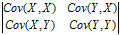协方差只能处理二维问题，即两个随机变量的相关程度。维数多了就需要计算多个协方差，于是出现了协方差矩阵。协方差矩阵的每一个值就是对应下标的两个随机变量的协方差（即相关程度）。
可以看出，协方差矩阵是一个对称矩阵，而且对角线是各个维度的方差。
python代码举例：

import numpy as np
X = np.array([[-2.1,-1,4.3],[3,1.1,0.12],[3,1.1,0.12]])
#每一行代表一个随机变量，每列代表随机变量的值
#[[-2.1,-1,4.3],
# [3,1.1,0.12],
# [3,1.1,0.12]]

print(np.cov(X))
#[[ 11.71      ,  -4.286     ,  -4.286     ],
# [ -4.286     ,   2.14413333,   2.14413333],
# [ -4.286     ,   2.14413333,   2.14413333]])

应用实例分析——机器学习中的分类问题
机器学习中的分类问题，给出178个葡萄酒样本，每个样本含有13个参数，比如酒精度、酸度、镁含量等，这些样本属于3个不同种类的葡萄酒。任务是提取3种葡萄酒的特征，以便下一次给出一个新的葡萄酒样本的时候，能根据已有数据判断出新样本是哪一种葡萄酒。
问题详细描述：http://archive.ics.uci.edu/ml/datasets/Wine训练样本数据：http://archive.ics.uci.edu/ml/machine-learning-databases/wine/wine.data
把数据集赋给一个178行13列（13个特征）的矩阵R，它的协方差矩阵C是13行13列的矩阵，对C进行特征分解，对角化其中U是特征向量组成的矩阵，D是特征组成的对角矩阵，并按由大到小排列。
然后，令：就实现了数据集在特征向量上的投影。
【注意】中的数据列是按照对应特征值的大小排列的，后面的列对应的特征值小，因而去掉以后对整个数据集的影响比较小。比如，现在我们通过上面的公式去掉后面的8列，只保留前5列（），就相当于将13维的数据通过PCA降到了5维。

Talk is cheap, show me the code!
根据蜥蜴书的内容，后面会专门开一个专栏分享如何使用sklearn库解决上述机器学习问题的code以及对应注释，同步会上传至Github上，敬请期待！

展开全文• 1、特征值是线性代数中的重要概念，设A是n阶方阵，如果存在数m非零n维列向量x，使得Ax=mx成立，则称m是A的一个特征值或本征值。 2、非零n维列向量x称为矩阵A的属于特征值m的特征向量或本征向量，简称A的特征向量。...线性代数
• 作者：阿狸 ...想要理解特征值，首先要理解矩阵相似。什么是矩阵相似呢？从定义角度就是：存在可逆矩阵P满足B＝则我们说AB是相似的。让我们来回顾一下之前得出的重要结论：对于同一个线性空间，数学之美
• 矩阵求特征值特征向量Java代码1.代码介绍2.参考文章 1.代码介绍   代码中实现的模块如下： 矩阵转置 点乘 自动索引生成 矩阵复制 获取给定索引矩阵所在行 矩阵行列式 矩阵正交化 矩阵求逆 矩阵QR分解(注：QR分解...QR算法 Java
• 雅可比法用于对称矩阵，QR用于非对称...QR分解法求特征向量及其特征值c++程序源于feitian_feiwu的资源，本资源增加了复特征值对应的特征向量计算。 vs2013下调试通过，复特征向量不唯一，程序里提供了在线验证网址，
• "------------------下面计算原始矩阵的特征值和特征向量-----------------------" eigenvalue , featurevector = np . linalg . eig ( X ) print "原始矩阵的特征值" print "eigenvalue=" , eigenvalue print...
• Hermite矩阵求特征值和特征向量的问题转化为求解实对称阵的特征值和特征向量
• 求矩阵的特征值，主要是用的QR分解，在我的有一次博客里，我已经详细地给出了计算的过程，大家有兴趣可以去看下，经过几天的钻研，终于完成了整个的eig算法。下面我将把我的整个代码附上，有不懂的可以问我，欢迎...c
• E=eig(A)：求解矩阵的全部特征值，构成向量E。 [X, D] = eig(A)：求矩阵A的全部特征值，构成对角阵D；并产生矩阵X，X各列是相应的特征向量。 2、代码示例 clc; clear all; A = [1,3,8;6,1,7;2,9,5]; %随便写的 ...
• ## 特征值与特征向量

万次阅读 2018-05-21 13:21:58
1. 什么是特征值？在数学上，特别是线性代数中，对于一个给定的矩阵，它的特征向量（eigenvector，也译固有向量或本征向量） 经过这个线性变换之后，得到的新向量仍然与原来的 保持在同一条直线上，但其长度或...
• QR法求解特征值特征向量(MATLAB)课程设计,已经调试成功，里面有4份不同的课设MATLAB
•机器学习 KL变换 深度学习
• 0.039147 1.698229 -0.825517 -0.035371 -0.632647 -1.157629 对称化,M1 = (M1+M1')/2 M1 = -1.20774 0.81459 0.62060 0.81459 1.69823 -0.72908 0.62060 -0.72908 -1.15763 计算特征向量和特征值, [V, LAMBDA] = ...
• 复数矩阵的特征值分解，使用了GSL科学计算函数库，使得特征值分解时间大大减少。
• 特征值与特征向量的几何意义（转） 2016年9月9日 ReidHolmes Comments 0 Comment   矩阵的乘法是什么，别只告诉我只是“前一个矩阵的行乘以后一个矩阵的列”，还会一点的可能还会说“前一个矩阵的列数...
• 特征值和特征向量——在矩阵的数值计算中不可或缺的一环，但大多数人仅满足于套用公式 f(λ)=∣λE−A∣f(\lambda)=\mid \lambda E-A\midf(λ)=∣λE−A∣ 止步于粗浅地求出相应的数值和向量. 在笔者看来未免太过...线性代数 机器学习
• MatrixXcd A(4，4); ComplexEigenSolver<MatrixXcd> es(A); B = es.eigenvalues(); C = es.eigenvectors(); cout << "eigenvalues is \n" << B <... "size of B is \n" ...eigen c++
• 计算方阵的特征值和右特征向量。 参数： a : ( …，M，M）数组 将计算特征值和右特征向量的矩阵 返回： w : ( …，M）数组 特征值，每个都根据其多样性重复。特征值不一定是有序的。结果数组将是复数类型，除非...
• 如果矩阵对某一个向量或某些向量只发生伸缩变换，不对这些向量产生旋转的效果，那么这些向量就称为这个矩阵的特征向量，伸缩的比例就是特征值。 实际上，上述的一段话既讲了矩阵变换特征值及特征向量的几何意义...
• 该程序求解了hermit矩阵的特征值和特征向量
• 如果矩阵对某一个向量或某些向量只发生伸缩变换，不对这些向量产生旋转的效果，那么这些向量就称为这个矩阵的特征向量，伸缩的比例就是特征值。 实际上，上述的一段话既讲了矩阵变换特征值及特征向量的几何意义...
• 1. 特征值与奇异值的主要区别 两者的主要区别在于：奇异值分解主要用于数据矩阵，而特征植分解主要用于方型的相关矩阵。...本征值和本征向量为量子力学术语，对矩阵来讲与特征值和特征向量定义一样。但本征
• 对称矩阵的特征值和特征向量 这一节，我们首先研究一类重要的矩阵，实对称矩阵，的特征值和特征向量。 性质 我们的主要结论是 实对称矩阵的特征值全部是实数。 实对称矩阵可以取到 nnn 个正交的特征实向量。 原因 ...
• 6.1 特征值与特征向量特征向量：若A为n阶方阵，如果存在一个非零向量x使得Ax=λxAx=\lambda x，则称标量λ\lambda为特征值(eigenvalue)，称x为属于λ\lambda的特征向量(eigenvector)。特征向量与零度空间：方程Ax=λ......